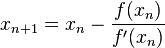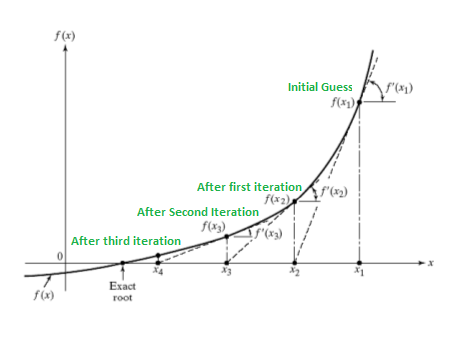Related Articles

# Program for Newton Raphson Method

• Difficulty Level : Medium
• Last Updated : 06 Apr, 2021

Given a function f(x) on floating number x and an initial guess for root, find root of function in interval. Here f(x) represents algebraic or transcendental equation.
For simplicity, we have assumed that derivative of function is also provided as input.
Example:

```Input: A function of x (for example x3 – x2 + 2),
derivative function of x (3x2 – 2x for above example)
and an initial guess x0 = -20
Output: The value of root is : -1.00
OR any other value close to root.```

We have discussed below methods to find root in set 1 and set 2
Set 1: The Bisection Method
Set 2: The Method Of False Position
Comparison with above two methods:

1. In previous methods, we were given an interval. Here we are required an initial guess value of root.
2. The previous two methods are guaranteed to converge, Newton Rahhson may not converge in some cases.
3. Newton Raphson method requires derivative. Some functions may be difficult to
impossible to differentiate.
4. For many problems, Newton Raphson method converges faster than the above two methods.
5. Also, it can identify repeated roots, since it does not look for changes in the sign of f(x) explicitly

The formula:
Starting from initial guess x1, the Newton Raphson method uses below formula to find next value of x, i.e., xn+1 from previous value xnAlgorithm:
Input: initial x, func(x), derivFunc(x)
Output: Root of Func()

1. Compute values of func(x) and derivFunc(x) for given initial x
2. Compute h: h = func(x) / derivFunc(x)
3. While h is greater than allowed error ε
1. h = func(x) / derivFunc(x)
2. x = x – h

Below is the implementation of above algorithm.

## C++

 `// C++ program for implementation of Newton Raphson Method for``// solving equations``#include``#define EPSILON 0.001``using` `namespace` `std;` `// An example function whose solution is determined using``// Bisection Method. The function is x^3 - x^2  + 2``double` `func(``double` `x)``{``    ``return` `x*x*x - x*x + 2;``}` `// Derivative of the above function which is 3*x^x - 2*x``double` `derivFunc(``double` `x)``{``    ``return` `3*x*x - 2*x;``}` `// Function to find the root``void` `newtonRaphson(``double` `x)``{``    ``double` `h = func(x) / derivFunc(x);``    ``while` `(``abs``(h) >= EPSILON)``    ``{``        ``h = func(x)/derivFunc(x);`` ` `        ``// x(i+1) = x(i) - f(x) / f'(x) ``        ``x = x - h;``    ``}` `    ``cout << ``"The value of the root is : "` `<< x;``}` `// Driver program to test above``int` `main()``{``    ``double` `x0 = -20; ``// Initial values assumed``    ``newtonRaphson(x0);``    ``return` `0;``}`

## Java

 `// Java program for implementation of``// Newton Raphson Method for solving``// equations``class` `GFG {``    ` `    ``static` `final` `double` `EPSILON = ``0.001``;``    ` `    ``// An example function whose solution``    ``// is determined using Bisection Method.``    ``// The function is x^3 - x^2 + 2``    ``static` `double` `func(``double` `x)``    ``{``        ``return` `x * x * x - x * x + ``2``;``    ``}``    ` `    ``// Derivative of the above function``    ``// which is 3*x^x - 2*x``    ``static` `double` `derivFunc(``double` `x)``    ``{``        ``return` `3` `* x * x - ``2` `* x;``    ``}``    ` `    ``// Function to find the root``    ``static` `void` `newtonRaphson(``double` `x)``    ``{``        ``double` `h = func(x) / derivFunc(x);``        ``while` `(Math.abs(h) >= EPSILON)``        ``{``            ``h = func(x) / derivFunc(x);``    ` `            ``// x(i+1) = x(i) - f(x) / f'(x)``            ``x = x - h;``        ``}``    ` `        ``System.out.print(``"The value of the"``                ``+ ``" root is : "``                ``+ Math.round(x * ``100.0``) / ``100.0``);``    ``}``    ` `    ``// Driver code``    ``public` `static` `void` `main (String[] args)``    ``{``        ` `        ``// Initial values assumed``        ``double` `x0 = -``20``;``        ``newtonRaphson(x0);``    ``}``}` `// This code is contributed by Anant Agarwal.`

## Python3

 `# Python3 code for implementation of Newton``# Raphson Method for solving equations` `# An example function whose solution``# is determined using Bisection Method.``# The function is x^3 - x^2 + 2``def` `func( x ):``    ``return` `x ``*` `x ``*` `x ``-` `x ``*` `x ``+` `2` `# Derivative of the above function``# which is 3*x^x - 2*x``def` `derivFunc( x ):``    ``return` `3` `*` `x ``*` `x ``-` `2` `*` `x` `# Function to find the root``def` `newtonRaphson( x ):``    ``h ``=` `func(x) ``/` `derivFunc(x)``    ``while` `abs``(h) >``=` `0.0001``:``        ``h ``=` `func(x)``/``derivFunc(x)``        ` `        ``# x(i+1) = x(i) - f(x) / f'(x)``        ``x ``=` `x ``-` `h``    ` `    ``print``(``"The value of the root is : "``,``                             ``"%.4f"``%` `x)` `# Driver program to test above``x0 ``=` `-``20` `# Initial values assumed``newtonRaphson(x0)` `# This code is contributed by "Sharad_Bhardwaj"`

## C#

 `// C# program for implementation of``// Newton Raphson Method for solving``// equations``using` `System;``class` `GFG {``    ` `    ``static` `double` `EPSILON = 0.001;``    ` `    ``// An example function whose solution``    ``// is determined using Bisection Method.``    ``// The function is x^3 - x^2 + 2``    ``static` `double` `func(``double` `x)``    ``{``        ``return` `x * x * x - x * x + 2;``    ``}``    ` `    ``// Derivative of the above function``    ``// which is 3*x^x - 2*x``    ``static` `double` `derivFunc(``double` `x)``    ``{``        ``return` `3 * x * x - 2 * x;``    ``}``    ` `    ``// Function to find the root``    ``static` `void` `newtonRaphson(``double` `x)``    ``{``        ``double` `h = func(x) / derivFunc(x);``        ``while` `(Math.Abs(h) >= EPSILON)``        ``{``            ``h = func(x) / derivFunc(x);``    ` `            ``// x(i+1) = x(i) - f(x) / f'(x)``            ``x = x - h;``        ``}``    ` `        ``Console.Write(``"The value of the"``                    ``+ ``" root is : "``                    ``+ Math.Round(x * 100.0) / 100.0);``    ``}``    ` `    ``// Driver code``    ``public` `static` `void` `Main ()``    ``{``        ` `        ``// Initial values assumed``        ``double` `x0 = -20;``        ``newtonRaphson(x0);``    ``}``}` `// This code is contributed by nitin mittal`

## PHP

 `= ``\$EPSILON``)``    ``{``        ``\$h` `= func(``\$x``) / derivFunc(``\$x``);` `        ``// x(i+1) = x(i) -``        ``// f(x) / f'(x)``        ``\$x` `= ``\$x` `- ``\$h``;``    ``}` `    ``echo` `"The value of the "``.``           ``"root is : "` `, ``\$x``;``}` `// Driver Code``\$x0` `= -20; ``// Initial values assumed``newtonRaphson(``\$x0``);` `// This code is contributed by ajit``?>`

## Javascript

 ``

Output:

`The value of root is : -1.00 `

How does this work?
The idea is to draw a line tangent to f(x) at point x1. The point where the tangent line crosses the x axis should be a better estimate of the root than x1. Call this point x2. Calculate f(x2), and draw a line tangent at x2We know that slope of line from (x1, f(x1)) to (x2, 0) is f'(x1)) where f’ represents derivative of f.

```f'(x1) = (0 - f(x1)) / (x2 - x1)

f'(x1) *  (x2 - x1) =  - f(x1)

x2 =  x1 - f(x1) / f'(x1)

By finding this point 'x2', we move closer towards the root.
We have to keep on repeating the above step till we get really close to
the root or we find it.

In general,
xn+1 =  xn - f(xn) / f'(xn) ```

Alternate Explanation using Taylor’s Series:

```Let x1 be the initial guess.

We can write x2 as below:
xn+1  = xn + h ------- (1)
Here h would be a small value that can be positive or negative.

According to Taylor's Series,
ƒ(x) that is infinitely differentiable can be written as below
f(xn+1) = f(xn  + h)
= f(xn) + h*f'(xn) + ((h*h)/2!)*(f''(xn)) + ...

Since we are looking for root of function, f(xn+1) = 0

f(xn) + h*f'(xn) + ((h*h)/2!)*(f''(xn)) + ... = 0

Now since h is small, h*h would be very small.
So if we ignore higher order terms, we get

f(xn) + h*f'(xn) = 0

Substituting this value of h = xn+1 - xn from equation (1) we get,
f(xn) + (xn+1  - xn)*f'(xn) = 0

xn+1 =  xn - f(xn) / f'(xn)  ```

Notes:

1. We generally used this method to improve the result obtained by either bisection method or method of false position.
2. Babylonian method for square root is derived from the Newton-Raphson method.

References:
Introductory Methods of Numerical Analysis by S.S. Sastry
https://en.wikipedia.org/wiki/Newton’s_method